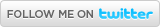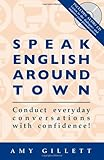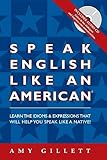Find meRecommended BooksA Lesson in Numbers

Friday, March 25, 2011 @ 02:03 PM

This is an English lesson which teaches how to pronounce numbers, including: ordinal numbers, fractions, decimals, dates, height, big numbers, telephone numbers, measurements and even the numbers that appear in the names of Kings and Queens. It explains the grammar rules we use for saying numbers and sentences with numbers as well as highlighting some irregularities.

Ordinal Numbers

Ordinal numbers are numbers used to indicate order, for example: 1st, 2nd, 3rd.

Most of them are formed by adding ‘th’ to the end of regular numbers, for example: fourth (four-th), sixth (six-th), seventh (seven-th). However, there are a few irregular ones: first (1st), second (2nd), third (3rd), fifth (5th), ninth (9th) and twelfth (12th). Here is a list of the ordinal numbers from 1st to 19th.

• 1st – first*
• 2nd – second*
• 3rd – third*
• 4th – fourth
• 5th – fifth*
• 6th – sixth
• 7th – seventh
• 8th – eighth
• 9th – ninth*
• 10th – tenth
• 11th – eleventh
• 12th – twelfth*
• 13th – thirteenth
• 14th – fourteenth
• 15th – fifteenth
• 16th – sixteenth
• 17th – seventeenth
• 18th – eighteenth
• 19th – nineteenth

For multiples of ten, the ‘y’ at end of the word changes to ‘ie’ and the suffix ‘eth’ is added.  For example, ‘twenty’ becomes ‘twentieth’. Here is a list of the multiples of ten from 20 to 90:

• 20th  - twentieth
• 30th – thirtieth
• 40th – fortieth
• 50th – fiftieth
• 60th – sixtieth
• 70th – seventieth
• 80th – eightieth
• 90th – ninetieth

Note: You say 100th as ‘one hundredth’.

Fractions

In order to say a fraction, we say the top number (numerator) as a regular number and we say the bottom number (denominator) as an ordinal number. For example, 1/8 is spoken as ‘one-eighth’.

If the top number is more than 1, then we pronounce the ordinal number as a plural. For example, 3/8 is spoken as ‘three-eighths. Here are some examples:

3/5 – three-fifths

7/12 – seven-twelfths

99/100 – ninety-nine one hundredths

There are two irregular fractions: half is used in place of second and quarter is used in place of fourth. For example, ½ is not said as ‘one-second’, it is called ‘one-half’, ‘a half’ or just ‘half’. Likewise, ¼ is not spoken as ‘one-fourth’, it is called ‘one-quarter’ or ‘a quarter’.

1 ½ - one and a half

2 ¼ - two and a quarter

More complex fractions are usually expressed in the following way:

218/713 – two hundred and eighteen over seven hundred and thirteen

Types of Fractions

3/4 hour – three quarters of an hour

7/10 mile – seven tenths of a mile

1/8 ton – one eighth of a ton

For fractions above 1, the terms of measurement is made plural:

1 ½ hours – one and a half hours

1 1/3 miles – one and a third miles

2 1/8 tons – two and one-eighth tons

Decimals

To pronounce decimals, we use the word ‘point’ for the dot and pronounce numbers one at a time. For example:

0.124 – nought point one two four (The figure 0 is zero in American English).

4.25 – four point two five

Types of Decimals

0.67m – nought point six seven metres (or nought point six seven of a metre)

0.3L – nought point three litres (or nought point three of a litre)

1.8kg – one point eight kilograms

Big Numbers

We do not make hundreds, thousands and millions plural when the number in front is more than 1. For example:

100 – one hundred

200 – two hundred

1000 – one thousand

4000 – four thousand

1,000 000 – one million

5,000 000 – five million

However, when there is no number in front, we do add an ‘s’. For example:

Hundreds of pounds

Thousands of eggs

Millions of dollars

The word ‘and’ is used between the hundreds and the tens in a number. For example:

420 – four hundred and twenty

2, 765 – two thousand, seven hundred and sixty-five

When the first figure is 1, we can say other ‘one’ or ‘a’. For example:

“He lived for a hundred years”

“You owe me one hundred dollars”

Telephone Numbers

Each number is pronounced separately unless there are two or three numbers next to each other that are the same. In this case, we might say ‘double’ or ‘triple’. For example:

258 7989 – two five eight seven nine eight nine

255 7989 – two double five seven nine eight nine

255 5989 – two triple five nine eight nine

In phone numbers, we usually pronounce the figure 0 as ‘oh’. For example:

205 7909 – two oh five seven nine oh nine

[The same rule applies to room numbers. For example if you were booked into room 102 of a hotel, you would pronounce it ‘one oh two’]

Dates

To pronounce the date within a month, we use ordinal numbers and the word ‘of’. For example:

15th March – fifteenth of March

12th September – twelfth of September

To pronounce a year before 2000 we usually pronounce it in two parts. For example:

1922 – 19/22 – nineteen twenty-two

1814 – 18/14 – eighteen fourteen

If there is a 0 in the ten position, we usually pronounce it ‘oh’. For example:

1908 – nineteen oh eight

1409 – fourteen oh nine

Compare to:

1980 – nineteen eighty

1490 – fourteen ninety

For years after 2000, we usually pronounce the whole number:

2002 – two thousand and two

2010 – two thousand and ten (a more informal way is to say twenty ten)

Height

Height can be said in centimetres or feet.

If we write in feet, a comma comes after the number of feet and quotations come after the number of inches. For example:

5’10 – five foot ten or (five feet ten inches)

6’3 – six foot three or (six feet three inches)

Notice that you can say either ‘foot’ or ‘feet’.

Here are some examples of height in centimetres:

163cm – one hundred and sixty-three centimetres

158cm – one hundred and fifty-eight centimetres

Kings and Queens

When Kings and Queens have numbers after their names, we pronounce them as ordinal numbers with the word ‘the’ in front. For example:

Henry VIII – Henry the eighth

Louis XII – Louis the twelfth